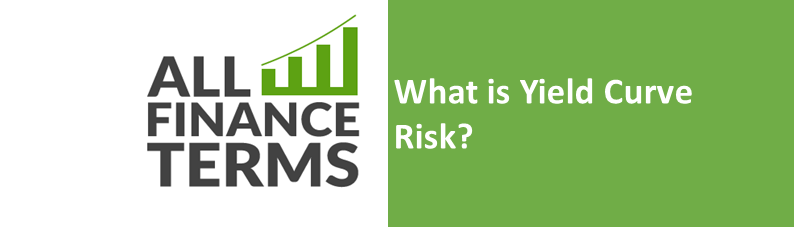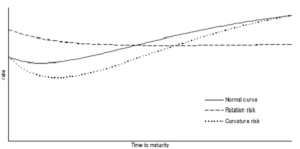## Definition of Yield Curve Risk

Yield curve risk also known as a rotation risk, is a risk of non-parallel shifts in the yield curve. It causes the curve to be steeper or flatter one. Curvature risk is also a type of yield curve risk in which the change in interest rate causes the curve to show more curvature.

## Explanation of Yield Curve Risk

Usually, when the maturity time is longer the interest rates are higher, therefore, the yield curve is upward sloping. The dashed line indicates rotation risk. We see that the long term rates decreased and short term rates increased and are resulting in a so called inverted yield curve.

The dotted line, we see is curvature risk. The long term and short term rates remain same as we see. Whereas, the medium term rates decrease, resulting in the yield curve, which shows more curvature, than the original one.## Relationship between Price and Yield

The price of a bond changes with the interest rates, change in the market. There is an inverse relationship between price and yield; lower bond price, high yield and high bond price, yields go down. Another important relation is the relationship between yield and maturity.

The yield curve is the graphical representation of that relationship in the market. If an investor or analyst is good at predicting changes in the yield curve, he or she will be able to benefit from the corresponding change in the prices of bonds.

Every bond portfolio has different exposures to how the yield curve shifts, i.e. yield curve risk. The predicted percentage change in a bond price that happens when yields fluctuation is captured by an advanced concept called “duration”